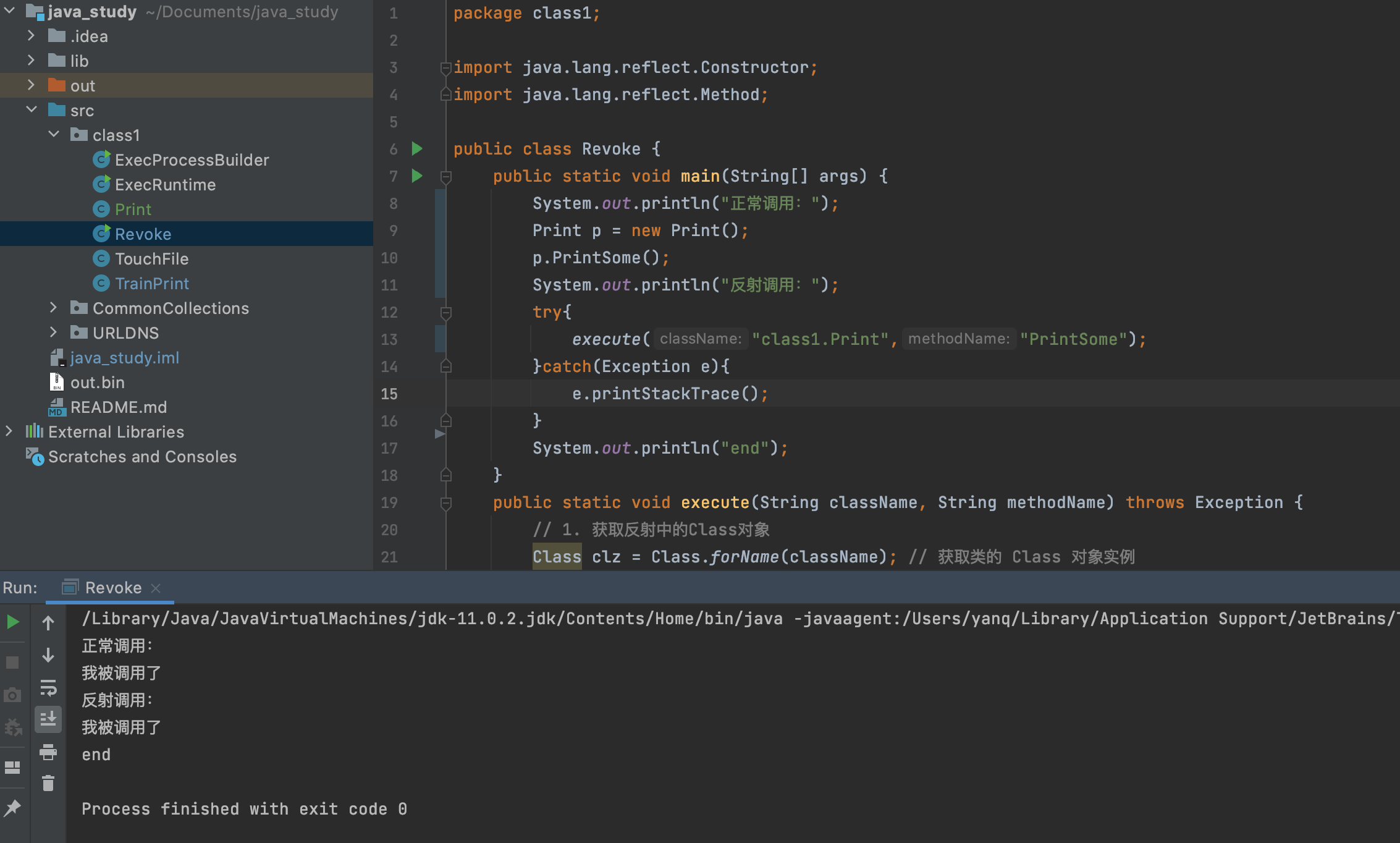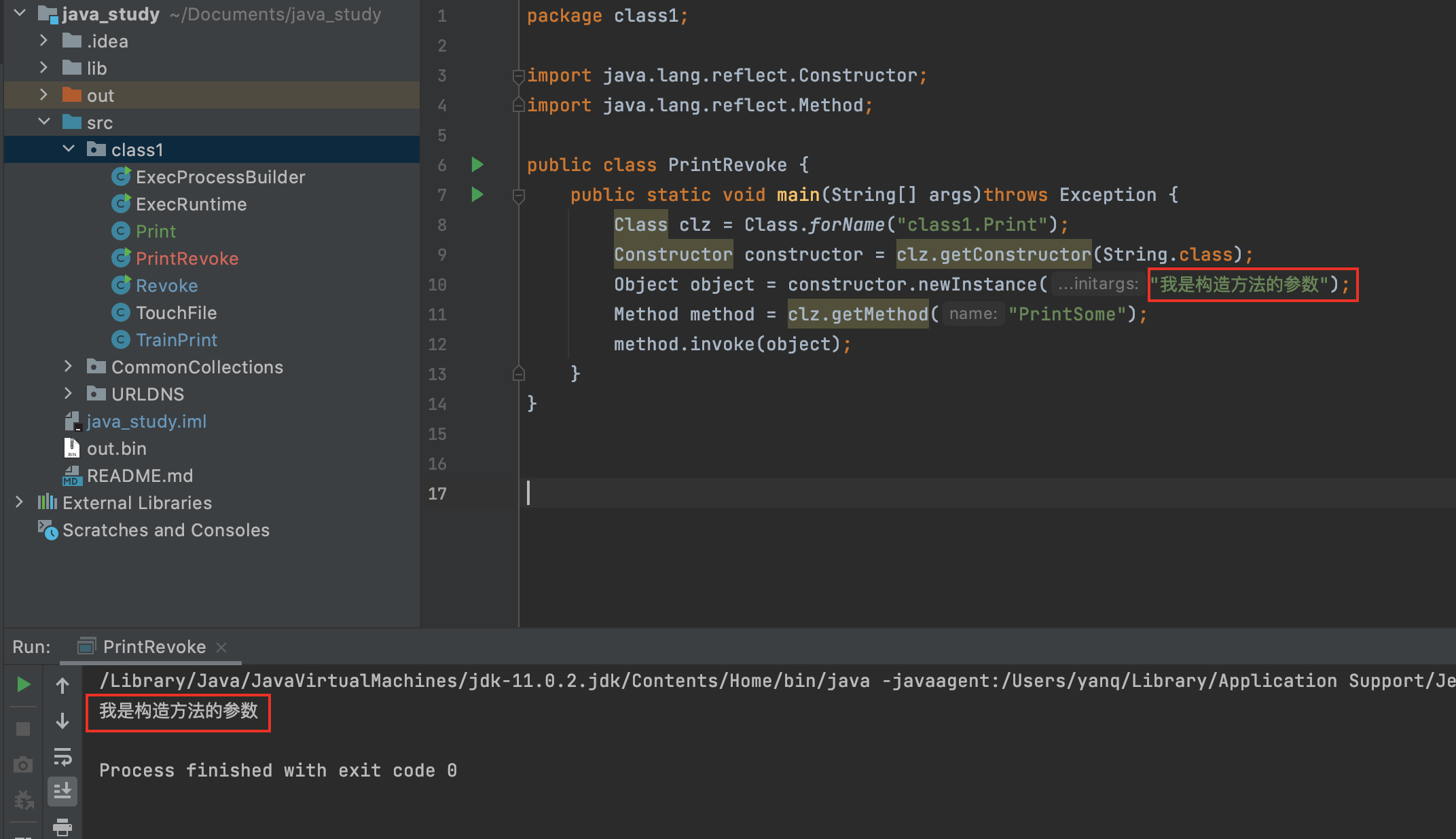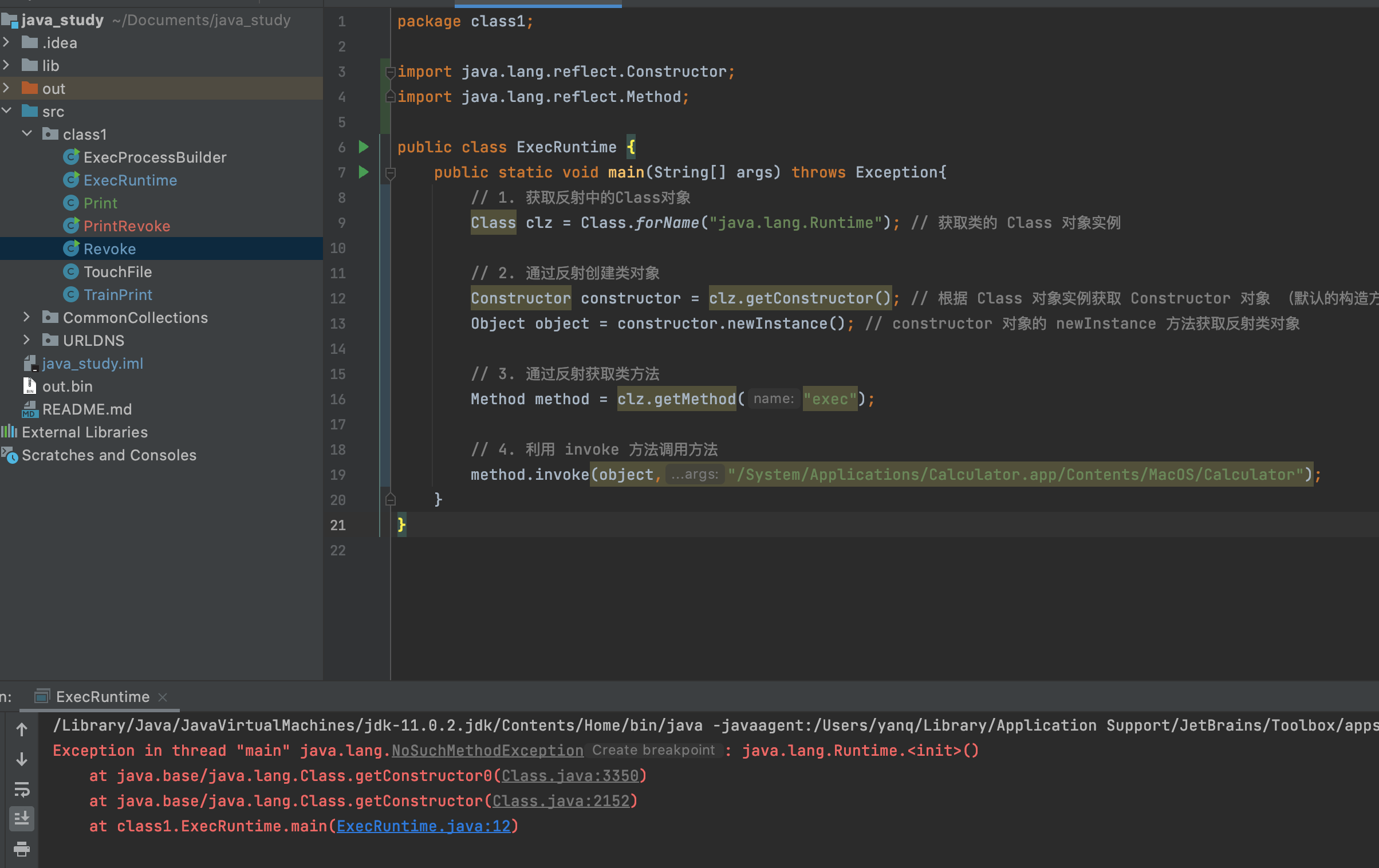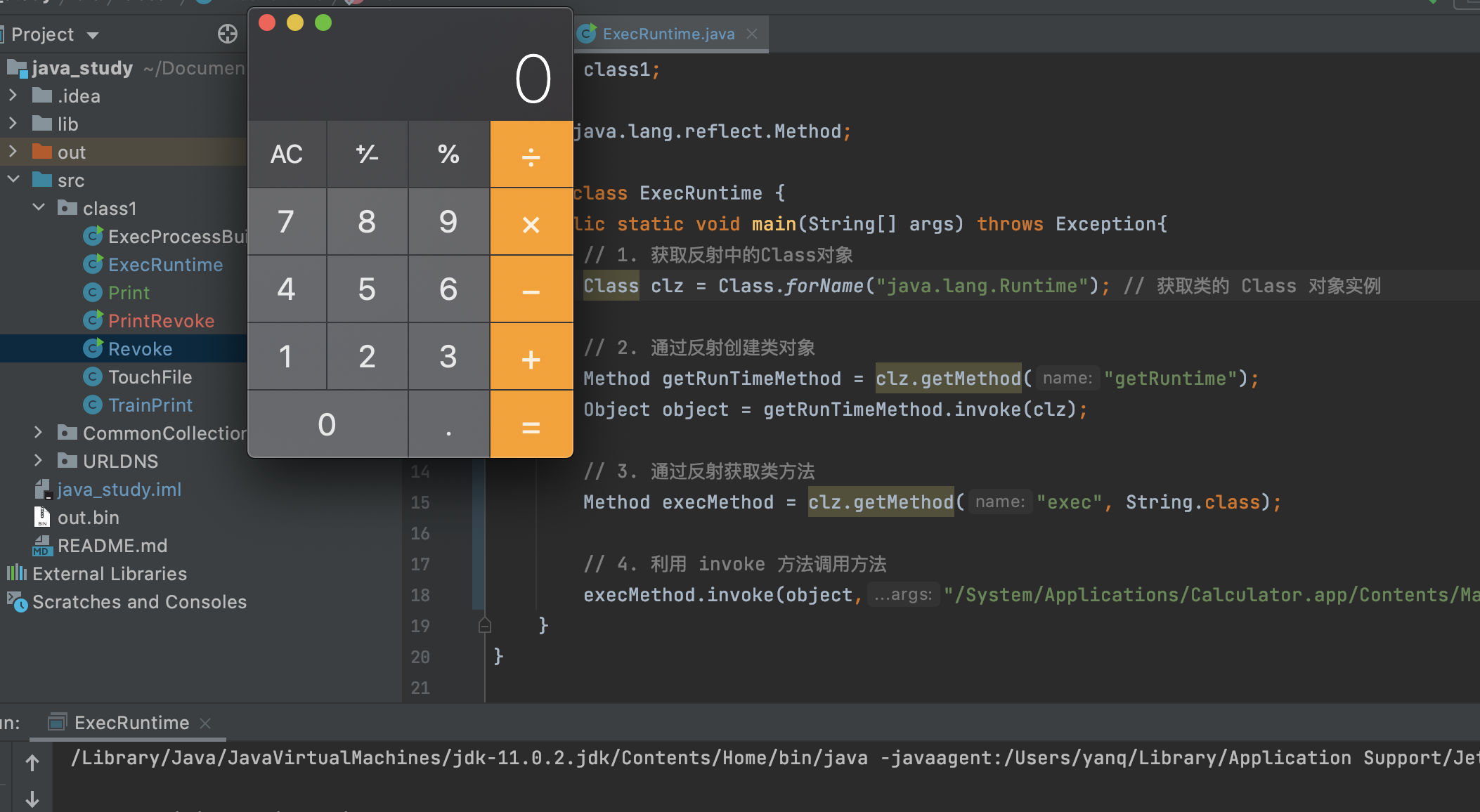# java 反射研究

## 1. 为什么需要java反射

java 代码在 new 某个类生成对象之前，都需要先 import 这个类，否则在编译时 jvm 会提示找不到这个类。

## 2. 代码实现java反射

``````package class1;

public class Print {
public String s ;
public Print(){
this.s = "我被调用了";
}
public Print(String st){
this.s = st;
}
public void PrintSome()  {
System.out.println(this.s);
}
}
``````

``````package class1;

import java.lang.reflect.Constructor;
import java.lang.reflect.Method;

public class Revoke {
public static void main(String[] args) {
System.out.println("正常调用：");
Print p = new Print();
p.PrintSome();
System.out.println("反射调用：");
try{
execute("class1.Print","PrintSome");
}catch(Exception e){
e.printStackTrace();
}
System.out.println("end");
}
public static void execute(String className, String methodName) throws Exception {
// 1. 获取反射中的Class对象
Class clz = Class.forName(className); // 获取类的 Class 对象实例

// 2. 通过反射创建类对象
Constructor constructor = clz.getConstructor(); // 根据 Class 对象实例获取 Constructor 对象 （默认的构造方法）
Object object = constructor.newInstance(); // constructor 对象的 newInstance 方法获取反射类对象

// 3. 通过反射获取类属性、方法、构造器
Method method = clz.getMethod(methodName);

// 4. 利用 invoke 方法调用方法
method.invoke(object);
}
}``````

``````正常调用：

end``````1. 通过类名获取 Class 对象
2. 通过反射创建类对象
3. 通过反射获取类属性、方法、构造器
4. 执行获取到的方法

### 2.1 通过类名获取Class对象

``Class clz = Class.forName("test1.Print");``

``Class clz = Print.class;``

obj.getClass() 来获取它的类

``````String str = new Print();
Class clz = str.getClass();``````

### 2.2 通过反射创建类对象

``````Class clz = Print.class;
Print p = (Print)clz.newInstance();``````

``````Class clz = Print.class;
Constructor constructor = clz.getConstructor();
Print p = (Print)constructor.newInstance();``````

``````package class1;

import java.lang.reflect.Constructor;
import java.lang.reflect.Method;

public class PrintRevoke {
public static void main(String[] args)throws Exception {
Class clz = Class.forName("class1.Print");
Constructor constructor = clz.getConstructor(String.class);
Object object = constructor.newInstance("我是构造方法的参数");
Method method = clz.getMethod("PrintSome");
method.invoke(object);
}
}``````### 2.3 通过反射获取类属性、方法、构造器

1.获取属性

``````// getFields() 方法可以获取 Class 类的公有属性
// getDeclaredFields() 方法则可以获取所有属性

Field[] fields = clz.getDeclaredFields();
for (Field field : fields) {
System.out.println(field.getName());
}``````

2.获取方法

``````// 获取当前类和父类中声明的公有方法，后面的参数是函数的参数类型列表。
Method method = clz.getMethod(String methodName,Class<?>... parameterTypes);

// 获取当前类中声明的所有方法
Method method = clz.getDeclaredMethod(methodName);   ``````

### 3.4 函数执行

``````Object invoke（Object obj,Object...args）
- obj：实例化后的对象
- args：用于方法调用的参数

## 3. 反射调用Runtime

``````package class1;

import java.lang.Runtime;

public class ExecRuntime {
public static void main(String[] args) throws Exception{
Runtime.getRuntime().exec("/System/Applications/Calculator.app/Contents/MacOS/Calculator");
}
}``````

``````package class1;

import java.lang.reflect.Constructor;
import java.lang.reflect.Method;

public class ExecRuntime {
public static void main(String[] args) throws Exception{
// 1. 获取反射中的Class对象
Class clz = Class.forName("java.lang.Runtime");

// 2. 通过反射创建类对象
Constructor constructor = clz.getConstructor(); // 根据 Class 对象实例获取 Constructor 对象 （默认的构造方法）
Object object = constructor.newInstance(); // constructor 对象的 newInstance 方法获取反射类对象

// 3. 通过反射获取类方法
Method method = clz.getMethod("exec", String.class);

// 4. 利用 invoke 方法调用方法
method.invoke(object,"/System/Applications/Calculator.app/Contents/MacOS/Calculator");
}
}``````1.可以通过使用getDeclaredConstructor方法获取私有构造方法，再将其私有的构造方法设置为公有。

``````package class1;

import java.lang.reflect.Constructor;
import java.lang.reflect.Method;

public class ExecRuntime {
public static void main(String[] args) throws Exception{
// 1. 获取反射中的Class对象
Class clz = Class.forName("java.lang.Runtime");

// 2. 通过反射创建类对象
Constructor constructor = clz.getDeclaredConstructor(); // getDeclaredConstructor可以获取私有的构造函数
constructor.setAccessible(true); // 通过setAccessible(true)可以打破私有方法访问限制，从而进行调用
Object object = constructor.newInstance();

// 3. 通过反射获取类方法
Method method = clz.getMethod("exec", String.class);

// 4. 利用 invoke 方法调用方法
method.invoke(object,"/System/Applications/Calculator.app/Contents/MacOS/Calculator");
}
}``````

2.可以通过getRuntime方法返回一个对象。

``````package class1;

import java.lang.reflect.Method;

public class ExecRuntime {
public static void main(String[] args) throws Exception{
// 1. 获取反射中的Class对象
Class clz = Class.forName("java.lang.Runtime"); // 获取类的 Class 对象实例

// 2. 通过反射创建类对象
Method getRunTimeMethod = clz.getMethod("getRuntime");
Object object = getRunTimeMethod.invoke(clz);

// 3. 通过反射获取类方法
Method execMethod = clz.getMethod("exec", String.class);

// 4. 利用 invoke 方法调用方法
execMethod.invoke(object,"/System/Applications/Calculator.app/Contents/MacOS/Calculator");
}
}``````1 + 6 =